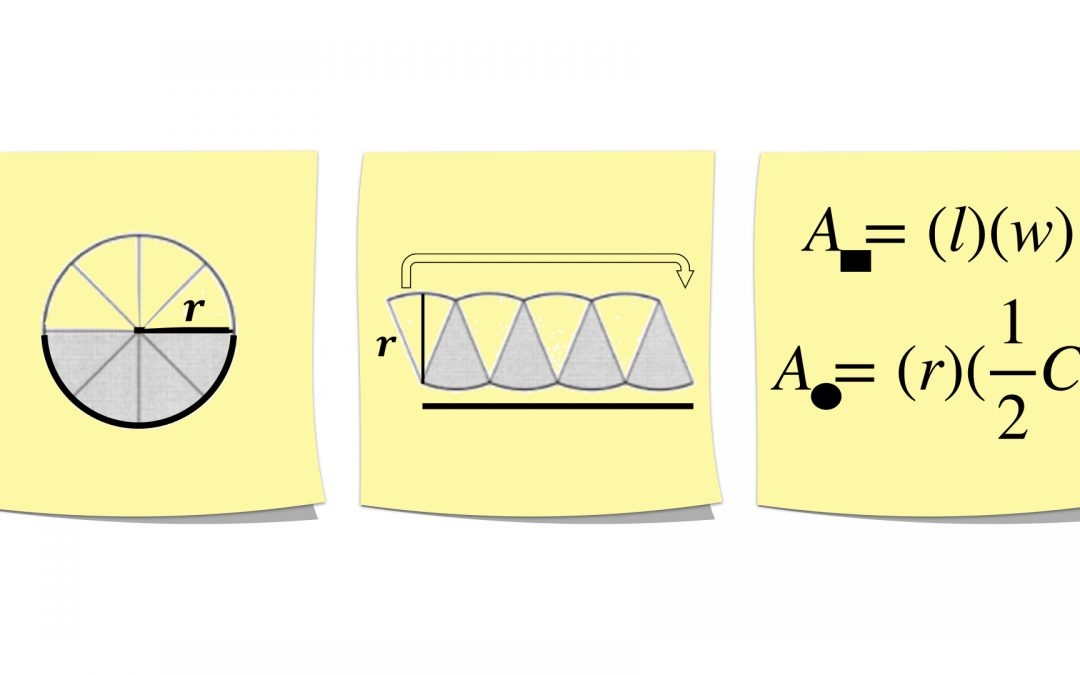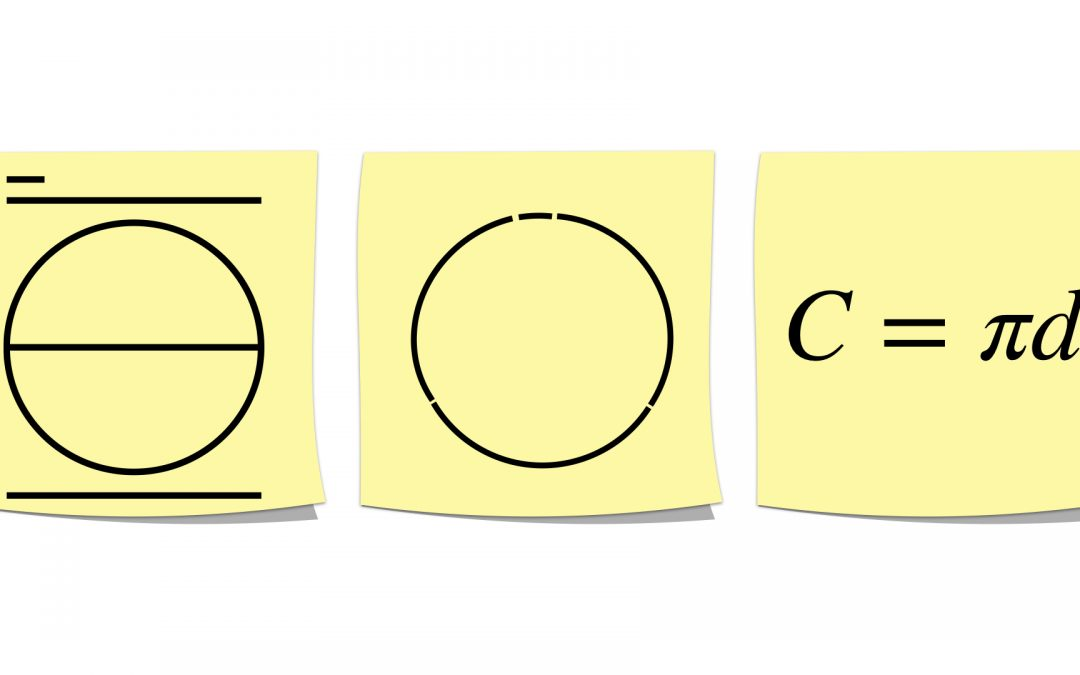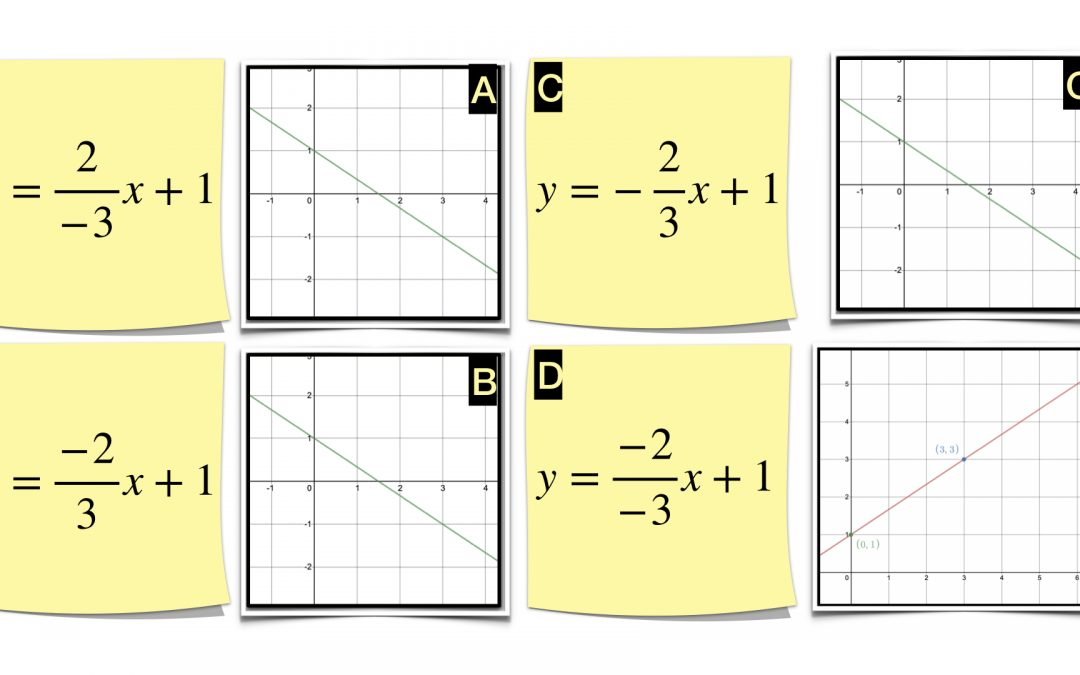Select Page## The Relationship between the Area of a Circle & its Circumference, Part Two: What does it Mean?

Common Core Standard: 7.G.B.4 In part one, I provided some Sticky Math Triads to put the process before students and teachers.  While I struggled there to provide visual representations, I believe I have accomplished the goal here as we examine the resulting...## The Relationship between the Area of a Circle and its Circumference, Part 1: Manipulating Formulas

Common Core Standard: 7.G.B.4 I have to confess that while I have done a lot of conceptual work with students around circumference and area of a circle (see some of it by checking out my blogposts on each subject), I have not done much to tackle the latter part of...## Area of a Circle Related to the Area of a Rectangle / Parallelogram

Common Core Standard: 7.G.B.4 If area is defined as the number of unit squares that cover the surface of a closed figure, then how does this apply to a circle? Can we remember the abstract formula for area of a circle better by understanding where it comes from...## Circumference of a Circle & the Meaning of Pi

Common Core Standard: 7.G.B.4 These Sticky Math activities can each stand alone; however, each of these blogposts suggests how you might put them together to form a lesson.  You can always adapt them to suit your needs. This Sticky Math Lesson helps build fluency from...

## Which One & Why?

Common Core Standards (different examples support different standards): 6.EE.A.2, 7.EE.A.1, 8.F.A.2, 8.F.A.3, HSA.SSE.A.1.A, HSF.IF.B.4 Besides using Sticky Math to compare two different representations or match representations, you could provide two nonequivalent...## Square Roots & the Birth of Sticky Math

Common Core Standards: 8.NS.A.2 . Disclaimer: This post includes data from estimating square roots, but focuses on the concept of a square root.  More on how to estimate square roots in a future blog post. I was working with a teacher who had just given a Smarter...## Negatives in a Slope Fraction

Common Core Standard: 8.F.A.2, 8.F.A.3 Use: Individually as a quick Sticky Math Warmup, in groups for a longer Sticky Math Warmup, or all together as a Sticky Math Lesson Note: Post It sells sticky notes with grids on them, which makes using Sticky Math with graphing...## Slope Intercept Form

Common Core Standard: 8.F.A.2, 8.F.A.3 Use: Individually as a quick Sticky Math Warmup, in groups of three for a longer Sticky Math Warmup, or all together as a Sticky Math Lesson Note: Post It sells sticky notes with grids on them, which makes using Sticky Math with...## Multiplication & the Distributive Property

Common Core Standard: 3.OA.B.5 A week’s’ worth of warm ups or a single lesson; the choice is yours. If you need to revisit for conceptual, interleaved practice to build fluency and retention, then use this as a week of warmups. Alternatively, you could use...## Fluently Add and Subtract within 10

1.OA.C.6 The Sticky Math Triad below asks for students to make connections between representations while beginning to explore the Commutative Property.  By asking students to create additional equivalent representations, students may choose to find the sum by counting...